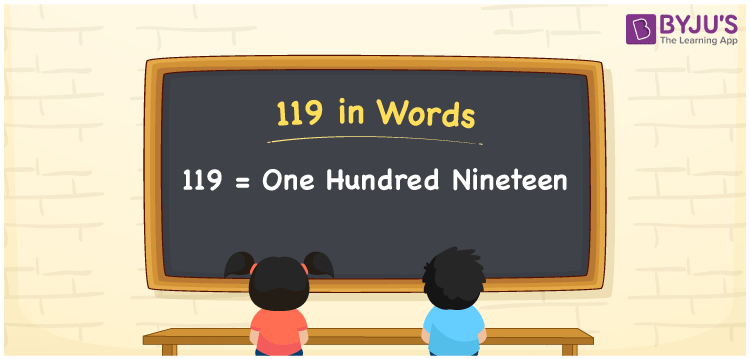# 119 in Words

119 in words is one hundred nineteen. The number 119 is a three-digit number and it is a cardinal number. For example, The cost of chocolate is Rs. 119. Learning the number and the number name is essential for everyone, which we use in our daily life. To convert numbers into words, the place value chart is very helpful. This article will help you to write the number 119 in words.

 119 in Words: One Hundred Nineteen. One Hundred Nineteen in Numerical Form: 119.

## 119 in English Words## How to Write 119 in Words?

The following is the place value table for the three-digit number 119.

 Hundreds Tens Ones 1 1 9

The expanded form of 119 is as follows:

= 1 × Hundred + 1 × Ten + 9 × One

= 1 × 100 + 1 × 10 + 9 × 1

= 100 + 10 + 9

= 119

= One hundred nineteen

Hence, 119 in words is one hundred nineteen.

119 in words – One hundred nineteen

Is 119 an odd number? – Yes

Is 119 an even number? – No

Is 119 a perfect square number? – No

Is 119 a perfect cube number? – No

Is 119 a prime number? – No

Is 119 a composite number? – Yes

## Frequently Asked Questions on 119 in Words

Q1

### How to write 119 in words?

119 in words is one hundred nineteen.

Q2

### Simplify 100 + 19, and express it in words.

Simplifying 100 + 19, we get 119. Hence, 119 in words is one hundred nineteen.

Q3

### How to write one hundred nineteen in numbers?

One hundred nineteen in numbers is 119.